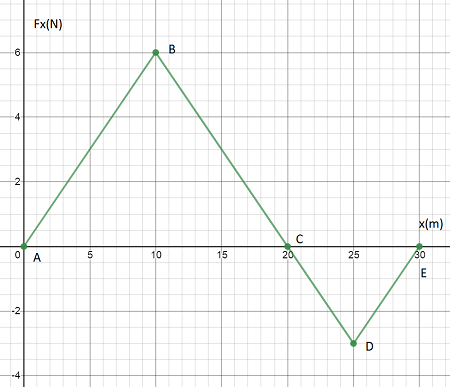# The force acting on a particle varies as shown in the figure below. (The x axis in the graph has...

## Question:

The force acting on a particle varies as shown in the figure below. (The x axis in the graph has its tick marks marked in increments of {eq}\displaystyle 5.00 \ m {/eq}.) Find the work done by the force on the particle as it moves across the following distances.

A.) from {eq}\displaystyle x = 0 \ m {/eq} to {eq}\displaystyle x = 20.00 \ m {/eq}

B.) from {eq}\displaystyle x = 20.00 \ m {/eq} to {eq}\displaystyle x = 30.00 \ m {/eq}

C.) from {eq}\displaystyle x = 0 \ m {/eq} to {eq}\displaystyle x = 30.00 \ m {/eq}## Work Done by a Variable Force:

For a force-displacement graph, the work done by the force for a displacement interval is simply the area under the graph for that displacement interval.

Become a Study.com member to unlock this answer! Create your account

#### Part A

The work-done, 'W1 in the displacement interval 0-20 m is the area of the triangle:

{eq}\begin{align*} W_1 &= \frac{1}{2}bh\\ &=...Work Done by a Variable Force

from

Chapter 8 / Lesson 4
13K

Doing work on an object is a simple concept: we apply a certain force over a certain distance. But in real life, that force is rarely constant. Therefore, we need to understand variable forces and be able to calculate them accurately.